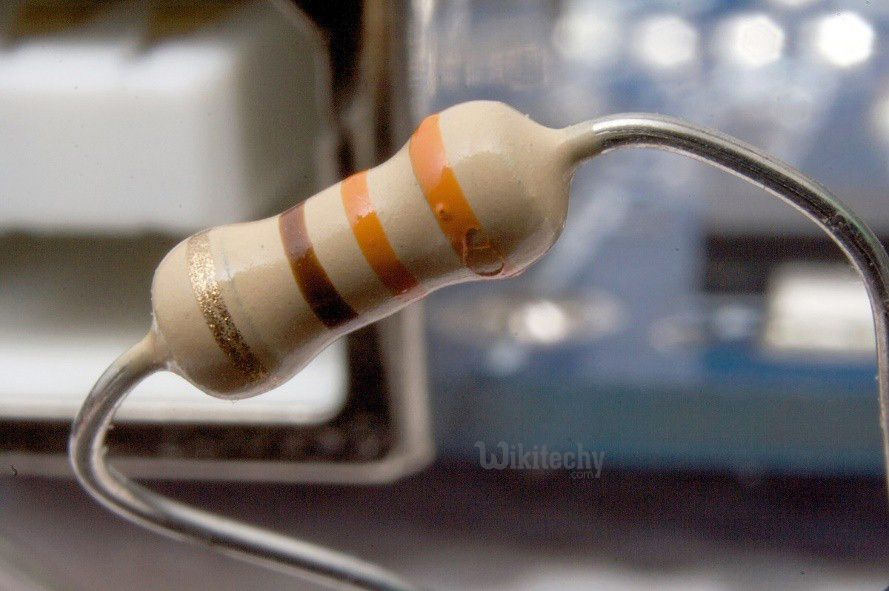# 555 Timer LED Flasher - What is Resistor ?

## Resistor

• Resistors are a specific, never-changing electrical resistance.
• Resistors are electronic components
• The resistor’s resistance restricts the flow of electrons through a circuit.
• Resistors are common elements of electrical networks and electronic circuits and are global in electronic equipment.
• Practical resistors as discrete components can be composed of various compounds and forms. Resistors are also implemented within integrated circuits.Resistor

• Variable resistors are called potentiometers
• There is a fixed value of resistance between two terminals
• The moving part of the potentiometer is called the wiper.
• Four band resistor colour code
• 1st band provides the first digit of the code
• 2nd band provides the second digit of the code
• 3rd band is the multiplier
• 4th band indicates the tolerance valueResistor

## Resistor colour code calculation

• The first band red has a value of 2
• The second band purple has a value of 7
• The third band has a multiplier of x 10
• The last band indicates a tolerance value of +/-5%
• Resistance value is 270Ω +/-5%

## Ohm's law

• The behaviour of an ideal resistor is dictated by the relationship specified by Ohm's law:
• Ohm's law states that the voltage (V) across a resistor is proportional to the current (I), where the constant of proportionality is the resistance (R).

## Series and parallel resistors

• The total resistance of resistors connected in series is the sum of their individual resistance values.Serial Resistors

• The total resistance of resistors connected in parallel is the reciprocal of the sum of the reciprocals of the individual resistors.parallel Resistor